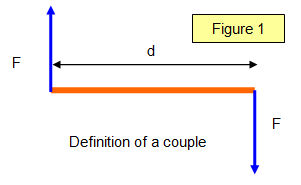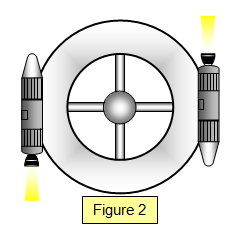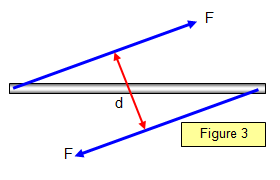# Couples

Every couple has its moment!If two equal and opposite forces whose lines of action are not the same act on a body then they produce only a turning effect on the body - such an effect is called a couple and the size of this couple is called the moment of the couple or torque. (See Figure 1) If these two forces are both equal and opposite the object will simply rotate but if they are not then the object will translate as well.

## DEFINITION OF A COUPLE

A couple is composed of two forces that:
(a) are equal
(b) are anti-parallel (parallel but in opposite directions)
(c) do not pass through the same point

The moment of a couple is called a torque. The size of the torque is given by :Torque = [Fxd/2]x2 = Fd

(Torques are measured in Nm).

A space station may be rotated by two rockets placed at opposite ends of a diagonal. They produce a couple which makes the space station spin. Two hands rotating the steering wheel of a car is a further example of a couple.

Example problems
1. Two forces of 20 N is applied to a car steering wheeel that has a diameter 40 cm. If the two forces act tangentially to the steering wheel and in antiparallel directions calculate the torque applied.

Torque = [Fxd/2]x2 = 20x0.2x2 = 8 Nm

2. Explain carefully what would happen if the two rockets in Figure 2 were not of equal thrust.

### Another look at couplesThe diagram shows that the distance between the lines of action of the forces is vital. As the forces are applied at different angles so the torque changes.

Torque = Fd

Think of trying to open a door by pulling on the handle so that your arm makes a very small angle with the door itself.

A VERSION IN WORD IS AVAILABLE ON THE SCHOOLPHYSICS USB